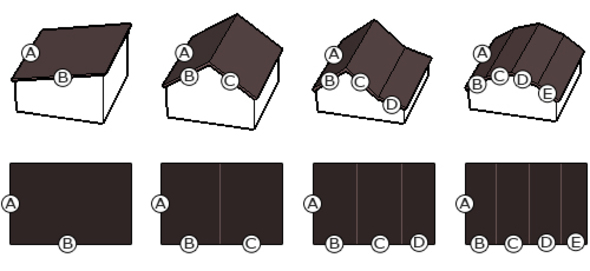44929
Physical

# Associated force of the hydraulic piston and the cylinder

Hydraulic cylinder is a mechanical akutatorem used for power transmission line by a linear thrust. Through the movement of the piston up and down over the forces and pressure. This calculator is used to calculate the two forces given angle for each operation.

Long description

see similar
55770
Physical

## Calculator resistance resistor ( bars)

Calculator gives the resistance of the resistor based on the introduced color bars.
53500
Physical

## Parallel connection of resistors

The calculator calculates the equivalent resistance of two resistors connected in parallel.
38705
Physical

## The energy stored in the capacitor

Calculator in supply capacitor, the capacitance and load resistance calculates the energy stored in the capacitor and the time constant of the RC circuit.
Users also viewed
805319
Building

## Insulation of wall

Jak policzyć izolację ścian?
Calculator allows you to calculate the amount of insulation panels needed to cover the walls of the building using the "light wet" or "dry light" methods.
218506
Financial

## ROCE - an indicator of the efficiency of investment

The calculator calculates ROCE on the basis of these pre-tax profits, total capital and current liabilities ROCE (called Return On Capital Employed) - an indicator of the efficiency and profitability of the investment, which is the capital of the company involved.
228573
Information technology

Calculator allows you to calculate the approximate download time on a given value for a circuit given parameters.
226899
Building

## Kalkulator powierzchni dachu

Jak obliczyć powierzchnię dachu?